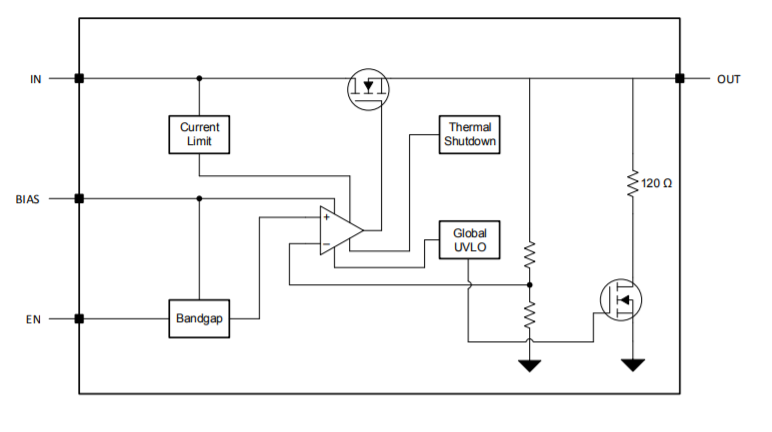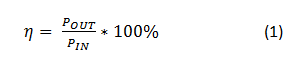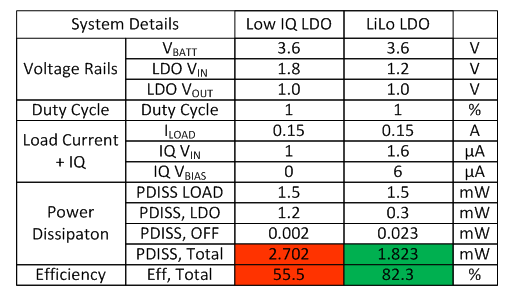# How LILO LDOs increase system efficiency

High efficiency for power supplies has historically been attributed to switching controllers or converters, whereas designers assume that linear regulators (LDOs) have bad efficiency. But linear regulator topologies have changed to where single n-channel p-channel n-channel (NPN)/p-channel n-channel p-channel (PNP) or p-channel metal-oxide semiconductor (PMOS)/n-channel MOS (NMOS) pass transistors can help achieve very low dropout voltages.

There have been three main power-supply trends in portable systems: decreasing bus voltages, compressed voltage conversions and decreasing quiescent current (IQ). These trends have led to the development of low-input low-output (LILO) LDOs.

As bus voltages have decreased, so have the minimum input voltage requirements for LDOs. As the bus rails dropped below 1.5V, the traditional LDO topology began reaching its limits because the input voltage rail powers all of the internal circuitry. The gradually decreasing input voltage led to the increased popularity of LILO LDOs.

LILO LDOs use an NMOS pass transistor and a bias rail to achieve low dropout. The advantage of using an NMOS pass transistor is that it has a lower drain-to-source resistance (RDS(on)) than a PMOS. It also needs a positive gate-to-source voltage (VGS) to operate. As a result of this topology, the bias rail is supplied by a higher voltage and powers most of the LDO’s internal circuitry, so the LDO can operate at lower input voltages.

One of the main benefits of the NMOS transistor is the low RDS(on), which allows for a smaller dropout per unit area than the PMOS transistor, enabling  smaller dropout voltages while maintaining a small size. Figure 1 shows the typical topology of an NMOS LDO from this diagram it can be seen that a Bias pin is required for this LDO to function properly.Figure 1: NMOS LDO Topology

As I said, the two advantages of LILO LDOs are lower input voltages and decreased dropout voltages. The latter advantage enables an increase in efficiency that is comparable to that of switch-mode power supplies. For all power supplies, you can calculate efficiency using Equation 1 as a function of the input and output power:For a LILO LDO like the TPS7A10, you can calculate the efficiency using Equation 2, since the LDO has both a bias rail and an input voltage rail:

If the load current is much greater than the IQ efficiency equation can be simplified, as shown in Equation 3:

You can see that the quickest way to increase efficiency in LDOs is to make the input and output voltage closer together by decreasing the dropout voltage.

In portable electronics, it is very common to have LDO-powered sensors because a switching converter generates too much noise. Designers will use low-IQ LDOs, believing that they increase the battery life of the system as the load is being pulsed. This is not necessarily the most efficient solution; however, as large power dissipation during the time where the load is on can cause drastically decreased efficiency.

Figure 2 shows two common power configurations for implementing a portable system. One uses a generic low-IQ LDO and the other uses a low-IQ LILO LDO. Comparing the power dissipation between the two solutions, the generic low-IQ LDO dissipates 2.7mW, while the LILO LDO dissipates 1.8mW (see Table 1). Using the LILO LDO increased the efficiency from 55% to 82%, even though the total IQ is higher on the LILO LDO.Figure 2: A low-IQ LDO vs. a LILO LDOTable 1: Efficiency calculations

As you can see, there is a clear benefit to implementing LILO LDOs in portable applications if battery life and efficiency are your main concerns. Reducing the differential between the input and output voltage enables these LDOs to achieve efficiencies greater than 80%. Once you understand this, you can select the proper LDO for your application.